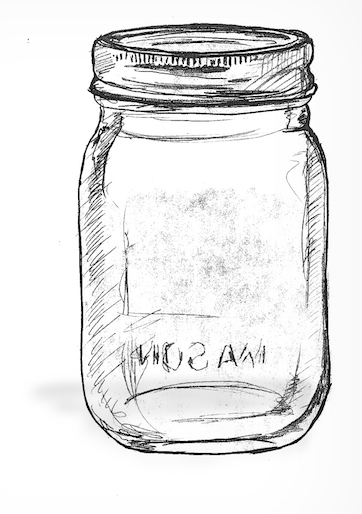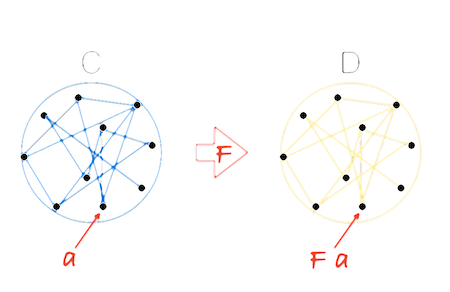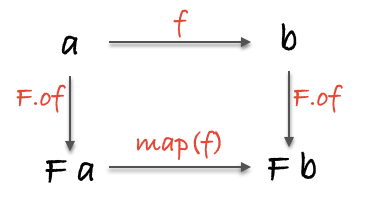# 特百惠

（譯者注：特百惠是美國家居用品品牌，代表產品是塑料容器。）

## 強大的容器``````var Container = function(x) {
this.__value = x;
}

Container.of = function(x) { return new Container(x); };
``````

``````Container.of(3)
//=> Container(3)

Container.of("hotdogs")
//=> Container("hotdogs")

Container.of(Container.of({name: "yoda"}))
//=> Container(Container({name: "yoda" }))
``````

• `Container` 是個只有一個屬性的物件。儘管容器可以有不止一個的屬性，但大多數容器還是隻有一個。我們很隨意地把 `Container` 的這個屬性命名為 `__value`
• `__value` 不能是某個特定的型別，不然 `Container` 就對不起它這個名字了。
• 資料一旦存放到 `Container`，就會一直待在那兒。我們可以`.__value` 獲取到資料，但這樣做有悖初衷。

## 第一個 functor

``````// (a -> b) -> Container a -> Container b
Container.prototype.map = function(f){
return Container.of(f(this.__value))
}
``````

``````Container.of(2).map(function(two){ return two + 2 })
//=> Container(4)

Container.of("flamethrowers").map(function(s){ return s.toUpperCase() })
//=> Container("FLAMETHROWERS")

Container.of("bombs").map(concat(' away')).map(_.prop('length'))
//=> Container(10)
``````

functor 是實現了 `map` 函式並遵守一些特定規則的容器型別。

## 薛定諤的 Maybe``````var Maybe = function(x) {
this.__value = x;
}

Maybe.of = function(x) {
return new Maybe(x);
}

Maybe.prototype.isNothing = function() {
return (this.__value === null || this.__value === undefined);
}

Maybe.prototype.map = function(f) {
return this.isNothing() ? Maybe.of(null) : Maybe.of(f(this.__value));
}
``````

`Maybe` 看起來跟 `Container` 非常類似，但是有一點不同：`Maybe` 會先檢查自己的值是否為空，然後才呼叫傳進來的函式。這樣我們在使用 `map` 的時候就能避免惱人的空值了（注意這個實現出於教學目的做了簡化）。

``````Maybe.of("Malkovich Malkovich").map(match(/a/ig));
//=> Maybe(['a', 'a'])

Maybe.of(null).map(match(/a/ig));
//=> Maybe(null)

//=> Maybe(null)

//=> Maybe(24)
``````

``````//  map :: Functor f => (a -> b) -> f a -> f b
var map = curry(function(f, any_functor_at_all) {
return any_functor_at_all.map(f);
});
``````

## 用例

``````//  safeHead :: [a] -> Maybe(a)
return Maybe.of(xs);
};

// Maybe(null)

``````

`safeHead` 與一般的 `_.head` 類似，但是增加了型別安全保證。引入 `Maybe` 會發生一件非常有意思的事情，那就是我們被迫要與狡猾的 `null` 打交道了。`safeHead` 函式能夠誠實地預告它可能的失敗——失敗真沒什麼可恥的——然後返回一個 `Maybe` 來通知我們相關資訊。實際上不僅僅是通知，因為畢竟我們想要的值深藏在 `Maybe` 物件中，而且只能通過 `map` 來操作它。本質上，這是一種由 `safeHead` 強制執行的空值檢查。有了這種檢查，我們才能在夜裡安然入睡，因為我們知道最不受人待見的 `null` 不會突然出現。類似這樣的 API 能夠把一個像紙糊起來的、脆弱的應用升級為實實在在的、健壯的應用，這樣的 API 保證了更加安全的軟體。

``````//  withdraw :: Number -> Account -> Maybe(Account)
var withdraw = curry(function(amount, account) {
return account.balance >= amount ?
Maybe.of({balance: account.balance - amount}) :
Maybe.of(null);
});

//  finishTransaction :: Account -> String
var finishTransaction = compose(remainingBalance, updateLedger); // <- 假定這兩個函式已經在別處定義好了

//  getTwenty :: Account -> Maybe(String)
var getTwenty = compose(map(finishTransaction), withdraw(20));

getTwenty({ balance: 200.00});

getTwenty({ balance: 10.00});
// Maybe(null)
``````

## 釋放容器裡的值

``````//  maybe :: b -> (a -> b) -> Maybe a -> b
var maybe = curry(function(x, f, m) {
return m.isNothing() ? x : f(m.__value);
});

//  getTwenty :: Account -> String
var getTwenty = compose(
maybe("You're broke!", finishTransaction), withdraw(20)
);

getTwenty({ balance: 200.00});

getTwenty({ balance: 10.00});
// "You're broke!"
``````

## “純”錯誤處理``````var Left = function(x) {
this.__value = x;
}

Left.of = function(x) {
return new Left(x);
}

Left.prototype.map = function(f) {
return this;
}

var Right = function(x) {
this.__value = x;
}

Right.of = function(x) {
return new Right(x);
}

Right.prototype.map = function(f) {
return Right.of(f(this.__value));
}
``````

`Left``Right` 是我們稱之為 `Either` 的抽象型別的兩個子類。我略去了建立 `Either` 父類的繁文縟節，因為我們不會用到它的，但你瞭解一下也沒壞處。注意看，這裡除了有兩個型別，沒別的新鮮東西。來看看它們是怎麼執行的：

``````Right.of("rain").map(function(str){ return "b"+str; });
// Right("brain")

Left.of("rain").map(function(str){ return "b"+str; });
// Left("rain")

Right.of({host: 'localhost', port: 80}).map(_.prop('host'));
// Right('localhost')

Left.of("rolls eyes...").map(_.prop("host"));
// Left('rolls eyes...')
``````

`Left` 就像是青春期少年那樣無視我們要 `map` 它的請求。`Right` 的作用就像是一個 `Container`（也就是 Identity）。這裡強大的地方在於，`Left` 有能力在它內部嵌入一個錯誤訊息。

``````var moment = require('moment');

//  getAge :: Date -> User -> Either(String, Number)
var getAge = curry(function(now, user) {
var birthdate = moment(user.birthdate, 'YYYY-MM-DD');
if(!birthdate.isValid()) return Left.of("Birth date could not be parsed");
return Right.of(now.diff(birthdate, 'years'));
});

getAge(moment(), {birthdate: '2005-12-12'});
// Right(9)

getAge(moment(), {birthdate: '20010704'});
// Left("Birth date could not be parsed")
``````

``````//  fortune :: Number -> String
var fortune  = compose(concat("If you survive, you will be "), add(1));

//  zoltar :: User -> Either(String, _)
var zoltar = compose(map(console.log), map(fortune), getAge(moment()));

zoltar({birthdate: '2005-12-12'});
// "If you survive, you will be 10"
// Right(undefined)

zoltar({birthdate: 'balloons!'});
// Left("Birth date could not be parsed")
``````

`Either` 並不僅僅只對合法性檢查這種一般性的錯誤作用非凡，對一些更嚴重的、能夠中斷程式執行的錯誤比如檔案丟失或者 socket 連線斷開等，`Either` 同樣效果顯著。你可以試試把前面例子中的 `Maybe` 替換為 `Either`，看怎麼得到更好的反饋。

``````//  either :: (a -> c) -> (b -> c) -> Either a b -> c
var either = curry(function(f, g, e) {
switch(e.constructor) {
case Left: return f(e.__value);
case Right: return g(e.__value);
}
});

//  zoltar :: User -> _
var zoltar = compose(console.log, either(id, fortune), getAge(moment()));

zoltar({birthdate: '2005-12-12'});
// "If you survive, you will be 10"
// undefined

zoltar({birthdate: 'balloons!'});
// "Birth date could not be parsed"
// undefined
``````

## 王老先生有作用...``````//  getFromStorage :: String -> (_ -> String)
var getFromStorage = function(key) {
return function() {
return localStorage[key];
}
}
``````

``````var IO = function(f) {
this.__value = f;
}

IO.of = function(x) {
return new IO(function() {
return x;
});
}

IO.prototype.map = function(f) {
return new IO(_.compose(f, this.__value));
}
``````

`IO` 跟之前的 functor 不同的地方在於，它的 `__value` 總是一個函式。不過我們不把它當作一個函式——實現的細節我們最好先不管。這裡發生的事情跟我們在 `getFromStorage` 那裡看到的一模一樣：`IO` 把非純執行動作（impure action）捕獲到包裹函式裡，目的是延遲執行這個非純動作。就這一點而言，我們認為 `IO` 包含的是被包裹的執行動作的返回值，而不是包裹函式本身。這在 `of` 函式裡很明顯：`IO(function(){ return x })` 僅僅是為了延遲執行，其實我們得到的是 `IO(x)`

``````//  io_window_ :: IO Window
var io_window = new IO(function(){ return window; });

io_window.map(function(win){ return win.innerWidth });
// IO(1430)

io_window.map(_.prop('location')).map(_.prop('href')).map(split('/'));
// IO(["http:", "", "localhost:8000", "blog", "posts"])

//  \$ :: String -> IO [DOM]
var \$ = function(selector) {
return new IO(function(){ return document.querySelectorAll(selector); });
}

// IO('I am some inner html')
``````

``````
////// 純程式碼庫: lib/params.js ///////

//  url :: IO String
var url = new IO(function() { return window.location.href; });

//  toPairs =  String -> [[String]]
var toPairs = compose(map(split('=')), split('&'));

//  params :: String -> [[String]]
var params = compose(toPairs, last, split('?'));

//  findParam :: String -> IO Maybe [String]
var findParam = function(key) {
return map(compose(Maybe.of, filter(compose(eq(key), head)), params), url);
};

////// 非純呼叫程式碼: main.js ///////

// 呼叫 __value() 來執行它！
findParam("searchTerm").__value();
// Maybe(['searchTerm', 'wafflehouse'])
``````

lib/params.js 把 `url` 包裹在一個 `IO` 裡，然後把這頭野獸傳給了呼叫者；一雙手保持的非常乾淨。你可能也注意到了，我們把容器也“壓棧”了，要知道建立一個 `IO(Maybe([x]))` 沒有任何不合理的地方。我們這個“棧”有三層 functor（`Array` 是最有資格成為 mappable 的容器型別），令人印象深刻。

``````var IO = function(f) {
this.unsafePerformIO = f;
}

IO.prototype.map = function(f) {
return new IO(_.compose(f, this.unsafePerformIO));
}
``````

`IO` 會成為一個忠誠的伴侶，幫助我們馴化那些狂野的非純操作。下一節我們將學習一種跟 `IO` 在精神上相似，但是用法上又千差萬別的型別。

## 非同步任務

``````// Node readfile example:
//=======================

var fs = require('fs');

err ? reject(err) : result(data);
});
});
};

// Task("One morning, as Gregor Samsa was waking up from anxious dreams, he discovered that
// in bed he had been changed into a monstrous verminous bug.")

// jQuery getJSON example:
//========================

//  getJSON :: String -> {} -> Task(Error, JSON)
var getJSON = curry(function(url, params) {
\$.getJSON(url, params, result).fail(reject);
});
});

getJSON('/video', {id: 10}).map(_.prop('title'));

// 傳入普通的實際值也沒問題
Task.of(3).map(function(three){ return three + 1 });
``````

`IO` 類似，`Task` 在我們給它綠燈之前是不會執行的。事實上，正因為它要等我們的命令，`IO` 實際就被納入到了 `Task` 名下，代表所有的非同步操作——`readFile``getJSON` 並不需要一個額外的 `IO` 容器來變純。更重要的是，當我們呼叫它的 `map` 的時候，`Task` 工作的方式與 `IO` 幾無差別：都是把對未來的操作的指示放在一個時間膠囊裡，就像家務列表（chore chart）那樣——真是一種精密的拖延術。

``````// Pure application
//=====================
// blogTemplate :: String

//  blogPage :: Posts -> HTML
var blogPage = Handlebars.compile(blogTemplate);

//  renderPage :: Posts -> HTML
var renderPage = compose(blogPage, sortBy('date'));

//  blog :: Params -> Task(Error, HTML)
var blog = compose(map(renderPage), getJSON('/posts'));

// Impure calling code
//=====================
blog({}).fork(
function(error){ \$("#error").html(error.message); },
function(page){ \$("#main").html(page); }
);

\$('#spinner').show();
``````

``````// Postgres.connect :: Url -> IO DbConnection
// runQuery :: DbConnection -> ResultSet

// Pure application
//=====================

//  dbUrl :: Config -> Either Error Url
var dbUrl = function(c) {
return (c.uname && c.pass && c.host && c.db)
? Right.of("db:pg://"+c.uname+":"+c.pass+"@"+c.host+"5432/"+c.db)
: Left.of(Error("Invalid config!"));
}

//  connectDb :: Config -> Either Error (IO DbConnection)
var connectDb = compose(map(Postgres.connect), dbUrl);

//  getConfig :: Filename -> Task Error (Either Error (IO DbConnection))
var getConfig = compose(map(compose(connectDB, JSON.parse)), readFile);

// Impure calling code
//=====================
getConfig("db.json").fork(
);
``````

## 一點理論

``````// identity
map(id) === id;

// composition
compose(map(f), map(g)) === map(compose(f, g));
``````

``````var idLaw1 = map(id);
var idLaw2 = id;

idLaw1(Container.of(2));
//=> Container(2)

idLaw2(Container.of(2));
//=> Container(2)
``````

``````var compLaw1 = compose(map(concat(" world")), map(concat(" cruel")));
var compLaw2 = map(compose(concat(" world"), concat(" cruel")));

compLaw1(Container.of("Goodbye"));
//=> Container('Goodbye cruel world')

compLaw2(Container.of("Goodbye"));
//=> Container('Goodbye cruel world')
````````````//  topRoute :: String -> Maybe(String)
var topRoute = compose(Maybe.of, reverse);

//  bottomRoute :: String -> Maybe(String)
var bottomRoute = compose(map(reverse), Maybe.of);

topRoute("hi");
// Maybe("ih")

bottomRoute("hi");
// Maybe("ih")
``````functor 也能巢狀使用：

``````var nested = Task.of([Right.of("pillows"), Left.of("no sleep for you")]);

map(map(map(toUpperCase)), nested);
// Task([Right("PILLOWS"), Left("no sleep for you")])
``````

`nested` 是一個將來的陣列，陣列的元素有可能是程式丟擲的錯誤。我們使用 `map` 剝開每一層的巢狀，然後對陣列的元素呼叫傳遞進去的函式。可以看到，這中間沒有回撥、`if/else` 語句和 `for` 迴圈，只有一個明確的上下文。的確，我們必須要 `map(map(map(f)))` 才能最終執行函式。不想這麼做的話，可以組合 functor。是的，你沒聽錯：

``````var Compose = function(f_g_x){
this.getCompose = f_g_x;
}

Compose.prototype.map = function(f){
return new Compose(map(map(f), this.getCompose));
}

var tmd = Task.of(Maybe.of("Rock over London"))

var ctmd = new Compose(tmd);

map(concat(", rock on, Chicago"), ctmd);
// Compose(Task(Maybe("Rock over London, rock on, Chicago")))

ctmd.getCompose;
// Task(Maybe("Rock over London, rock on, Chicago"))
``````

## 練習

``````require('../../support');
var _ = require('ramda');

// 練習 1
// ==========
// 使用 _.add(x,y) 和 _.map(f,x) 建立一個能讓 functor 裡的值增加的函式

var ex1 = undefined

//練習 2
// ==========
var xs = Identity.of(['do', 'ray', 'me', 'fa', 'so', 'la', 'ti', 'do']);

var ex2 = undefined

// 練習 3
// ==========
// 使用 safeProp 和 _.head 找到 user 的名字的首字母
var safeProp = _.curry(function (x, o) { return Maybe.of(o[x]); });

var user = { id: 2, name: "Albert" };

var ex3 = undefined

// 練習 4
// ==========
// 使用 Maybe 重寫 ex4，不要有 if 語句

var ex4 = function (n) {
if (n) { return parseInt(n); }
};

var ex4 = undefined

// 練習 5
// ==========
// 寫一個函式，先 getPost 獲取一篇文章，然後 toUpperCase 讓這片文章標題變為大寫

// getPost :: Int -> Future({id: Int, title: String})
var getPost = function (i) {
setTimeout(function(){
res({id: i, title: 'Love them futures'})
}, 300)
});
}

var ex5 = undefined

// 練習 6
// ==========
// 寫一個函式，使用 checkActive() 和 showWelcome() 分別允許訪問或返回錯誤

var showWelcome = _.compose(_.add( "Welcome "), _.prop('name'))

var checkActive = function(user) {
return user.active ? Right.of(user) : Left.of('Your account is not active')
}

var ex6 = undefined

// 練習 7
// ==========
// 寫一個驗證函式，檢查引數是否 length > 3。如果是就返回 Right(x)，否則就返回
// Left("You need > 3")

var ex7 = function(x) {
return undefined // <--- write me. (don't be pointfree)
}

// 練習 8
// ==========
// 使用練習 7 的 ex7 和 Either 構造一個 functor，如果一個 user 合法就儲存它，否則
// 返回錯誤訊息。別忘了 either 的兩個引數必須返回同一型別的資料。

var save = function(x){
return new IO(function(){
console.log("SAVED USER!");
return x + '-saved';
});
}

var ex8 = undefined
``````